International
Tables for
Crystallography
Volume B
Reciprocal space
Edited by U. Shmueli

International Tables for Crystallography (2006). Vol. B. ch. 2.5, pp. 286-287   | 1 | 2 |

## Section 2.5.3.2.1.  Direct and reciprocity symmetries: types I and II

P. Goodmanb

#### 2.5.3.2.1. Direct and reciprocity symmetries: types I and II

| top | pdf |

Convergent-beam diffraction symmetries are those of Schrödinger's equation, i.e. of crystal potential, plus the diffracting electron. The appropriate equation is given in Section 2.5.2[equation (2.5.2.6)] and Chapter 5.2 [equation (5.2.2.1)]in terms of the real-space wavefunction ψ. The symmetry elements of the crystal responsible for generating pattern symmetries may be conveniently classified as of two types (I and II) as follows.

• I. The direct (type I: Table 2.5.3.1) symmetries imposed by this equation on the transmitted wavefunction given z-axis illumination (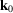, the incident wavevector parallel to Z, the surface normal) are just the symmetries of ϕ whose operation leaves both crystal and z axis unchanged. These are also called vertical' symmetry elements, since they contain Z. These symmetries apply equally in real and reciprocal space, since the operator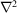has circular symmetry in both spaces and does nothing to degrade the symmetry in transmission. Hence, for high-symmetry crystals (zone axis parallel to z axis), and to a greater or lesser degree for crystals of a more general morphology, these zone-axis symmetries apply both to electron-microscope lattice images and to convergent-beam patterns under z-axis-symmetrical illumination, and so impact also on space-group determination by means of high-resolution electron microscopy (HREM). In CBED, these elements lead to whole pattern symmetries, to which every point in the pattern contributes, regardless of diffraction order and Laue zone (encompassing ZOLZ and HOLZ reflections).

 Table 2.5.3.1| top | pdf | Listing of the symmetry elements relating to CBED patterns under the classifications of vertical' (I), horizontal' (II) and combined or roto-inversionary axes
I. Vertical symmetry elements
International symbols
II. Horizontal symmetry elements
Diperiodic symbols BESR symbols
2′ m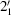m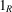a′, b′, n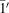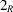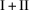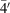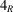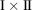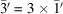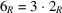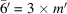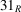• II. Reciprocity-induced symmetries, on the other hand, depend upon ray paths and path reversal, and in the present context have relevance only to the diffraction pattern. Crystal-inverting or horizontal crystal symmetry elements combine with reciprocity to yield indirect pattern symmetries lacking a one-to-one real-space correspondence, within individual diffraction discs or between disc pairs. Type II elements are assumed to lie on the central plane of the crystal, midway between surfaces, as symmetry operators; this assumption amounts to a central plane' approximation, which has a very general validity in space-group-determination work (Goodman, 1984a).

A minimal summary of basic theoretical points, otherwise found in Chapter 5.2and numerous referenced articles, is given here.

For a specific zero-layer diffraction order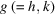the incident and diffracted vectors areand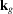. Then the three-dimensional vector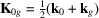has the pattern-space projection,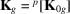. The point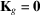gives the symmetrical Bragg condition for the associated diffraction disc, and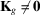is identifiable with the angular deviation of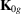from the vertical z axis in three-dimensional space (see Fig. 2.5.3.1).also defines the symmetry centre within the two-dimensional disc diagram (Fig. 2.5.3.2); namely, the intersection of the lines S and G, given by the trace of excitation error,, and the perpendicular line directed towards the reciprocal-space origin, respectively. To be definitive it is necessary to index diffracted amplitudes relating to a fixed crystal thickness and wavelength, with both crystallographic and momentum coordinates, as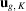, to handle the continuous variation of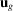(for a particular diffraction order), with angles of incidence as determined by, and registered in the diffraction plane as the projection of.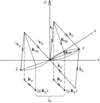Figure 2.5.3.1 | top | pdf |Vector diagram in semi-reciprocal space, using Ewald-sphere constructions to show the incident', reciprocity' and `reciprocity × centrosymmetry' sets of vectors. Dashed lines connect the full vectorsto their projections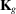in the plane of observation.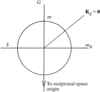Figure 2.5.3.2 | top | pdf |Diagrammatic representation of a CBED disc with symmetry lines m, mR (alternate labels G, S) and the central point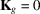.

### ReferencesGoodman, P. (1984a). A matrix basis for CBED pattern analysis. Acta Cryst. A40, 522–526.Google Scholar